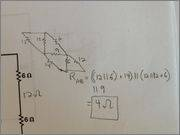# Circuit Simplification (Equivalent Resistance)

Bcranger 0

## Homework Statement

Determine the equivalent resistance of this circuit.

http://img444.imageshack.us/img444/5137/19910965.png [Broken]

## The Attempt at a Solution

So I realize that both of the 6 ohm resistors on each side are in series with each other. However, I am stuck after that part since I do not recognize a series or parallel connection from that point onwards. This is really in part due to the empty stretch of wire connecting the left and right vertical wires to the middle two wires. There are obviously no other resistors the 12 ohm equivalent resistors can connect to; there are also no two nodes that are shared by the 12 ohm equivalent resistor and another resistor.

Any clue as to how to proceed would be greatly appreciated.

Last edited by a moderator:

azizlwl
There are 2 vertical shorted connections.
You can see it clearer if you reduce each length until it becomes a point.

Bcranger 0
Right so I got an answer of 4 ohms as the equivalent resistance. Anyone concur?

azizlwl

Bcranger 0
Right, so first I combined the 6ohm resistors on each side into 12 ohm resistors. Then I redrew the circuit into deltas/triangles. This allowed me to find Rab by taking the 12 ohm on the right in parallel with the 6 ohm on the bottom half of the midsection. Then I took that equivalent resistor in series with the 14 ohm resistor. Then I took that entire equivalent resistor computed so far in parallel with the 9 ohm resistor and also (12 ohm left resistor in parallel with the 12 ohm top midsection resistor and then that equivalence in series with the top 6 ohm resistor).

Bcranger 0azizlwl